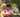### MaxSliceSum

February 10, 2020

MaxSliceSum

A non-empty array A consisting of N integers is given. A pair of integers (P, Q), such that 0 ≤ P ≤ Q < N, is called a slice of array A. The sum of a slice (P, Q) is the total of A[P] + A[P+1] + ... + A[Q].

Write a function:
function solution(A); that, given an array A consisting of N integers, returns the maximum sum of any slice of A.
For example, given array A such that:
A = 3 A = 2 A = -6
A = 4 A = 0
the function should return 5 because:
• (3, 4) is a slice of A that has sum 4,
• (2, 2) is a slice of A that has sum −6,
• (0, 1) is a slice of A that has sum 5,
• no other slice of A has sum greater than (0, 1).

Write an efficient algorithm for the following assumptions:
• N is an integer within the range [1..1,000,000];
• each element of array A is an integer within the range [−1,000,000..1,000,000];
• the result will be an integer within the range [−2,147,483,648..2,147,483,647].

``````function solution(A) {
// write your code in JavaScript (Node.js 8.9.4)
let max = A
let sum = 0
for(let i=0; i<A.length; i++){
sum += A[i]
if(sum>max) max = sum
if(sum<0) sum = 0
}

return max
}``````Posted by Tai Lu ( 呂台生 ) who's building useful things.You should follow him on Twitter